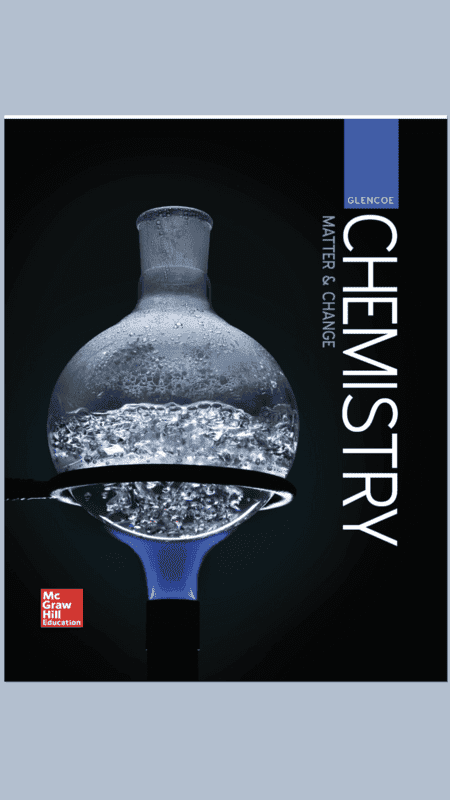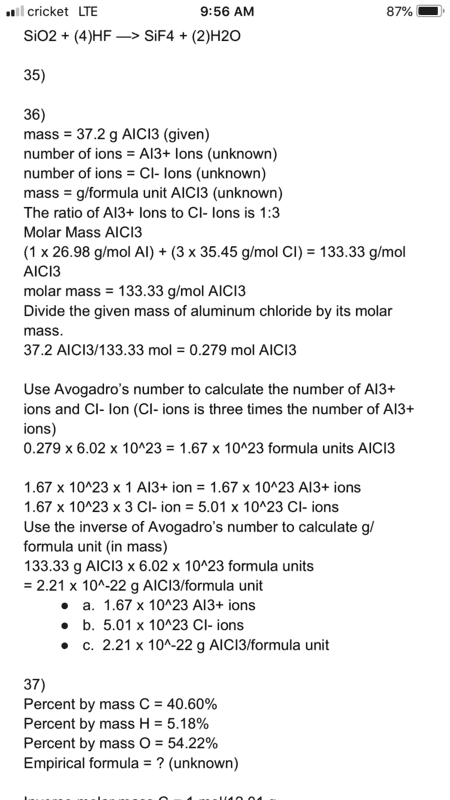# Mass to moles to particles

No. You would multiply the stoichiometric ratio by the number of moles given (or calculated in your case).
0.279 * 1:3 ratio?

Homework Helper
Gold Member
0.279 * 1:3 ratio?
What textbook are you using for class?

What textbook are you using for class?Homework Helper
Gold Member
Go to chapter 12 and review stiochiometric ratios used in calculations. In your example the ratio 1:3 would be the ratio of AlCl3 to Cl- and the ratio 3:1 would be the ratio of Cl- to AlCl3. You have to lnow when to use either one of them. Units inform that choice.
From this point you must show your units in calculations so you don’t use the wrong ratio or conversion factor.

Homework Helper
Gold Member
1 mole = 6.02 x 10^23 atoms

So how many Al atoms or ions does the 0.279 mol (which you calculated) contain?

Pretty easy question, one of us must have misunderstood what the question is.

So how many Al atoms or ions does the 0.279 mol (which you calculated) contain?

Pretty easy question, one of us must have misunderstood what the question is.
I thought the answer for part a and part b was 1 mole of aluminum ions and 3 moles of Chloride ions

Go to chapter 12 and review stiochiometric ratios used in calculations. In your example the ratio 1:3 would be the ratio of AlCl3 to Cl- and the ratio 3:1 would be the ratio of Cl- to AlCl3. You have to lnow when to use either one of them. Units inform that choice.
From this point you must show your units in calculations so you don’t use the wrong ratio or conversion factor.
I can’t find the chapter

Would it be 1.67 x 10^23?

Homework Helper
Gold Member
1 mole = 6.02 x 10^23 atoms
OK let's consider AlCl3 to be a molecule.
From the above quote, how many molecules of AlCl3 are there in 0.279 mol?

How many molecules of anything are there in 0.279 moles of it?

OK let's consider AlCl3 to be a molecule.
From the above quote, how many molecules of AlCl3 are there in 0.279 mol?

How many molecules of anything are there in 0.279 moles of it?
Am I suppose to multiply 6.02 x 10^23 by the number of moles?

Homework Helper
Gold Member
Am I suppose to multiply 6.02 x 10^23 by the number of moles?

Do you have no way of working out whether that is so or not except someone tells you? if you do that multiplication what will it tell you? OK a number, but what is it the number of and why?

I just have to guess why such an easy problem is holding you up so. One possibility is you have not understood what question they are asking. More probably or additionally it is a very common one found in this section again and again for questions of stoichiometry: that students have done many exercises in elementary school on something called "simple proportions". Only the lessons then were called "arithmetic" and they were self-contained excercises to get right but maybe not much idea given they would ever be any use for anything. When the same sort of calculation is called "chemistry" they can't do the calculations they did 10 years earlier any more or don't know what they mean.

Do you have no way of working out whether that is so or not except someone tells you? if you do that multiplication what will it tell you? OK a number, but what is it the number of and why?

I just have to guess why such an easy problem is holding you up so. One possibility is you have not understood what question they are asking. More probably or additionally it is a very common one found in this section again and again for questions of stoichiometry: that students have done many exercises in elementary school on something called "simple proportions". Only the lessons then were called "arithmetic" and they were self-contained excercises to get right but maybe not much idea given they would ever be any use for anything. When the same sort of calculation is called "chemistry" they can't do the calculations they did 10 years earlier any more or don't know what they mean.
I’ve found an example and attempted it. Hopefully everything is correct.
0.279 x 6.02 x 10^23 = 1.67 x 10^23 formula units AICI3

1.67 x 10^23 x 1 AI3+ ion = 1.67 x 10^23 AI3+ ions
1.67 x 10^23 x 3 CI- ion = 5.01 x 10^23 CI- ionsHomework Helper
Gold Member
That's right, it's not a guess or that way arbitrarily .

What does the new printed extract correspond to - worked solution in your textbook?

That's right, it's not a guess or that way arbitrarily .

What does the new printed extract correspond to - worked solution in your textbook?
What does that mean?

Homework Helper
Gold Member
What does that mean?
I just wondered what it meant after working through this problem for two pages you produce a solution that looked from print style like textbook extract. What is #48?